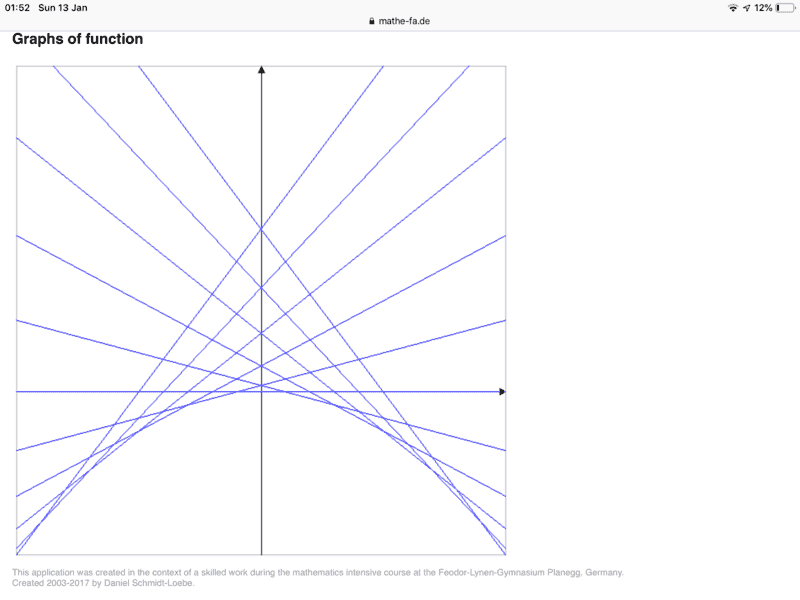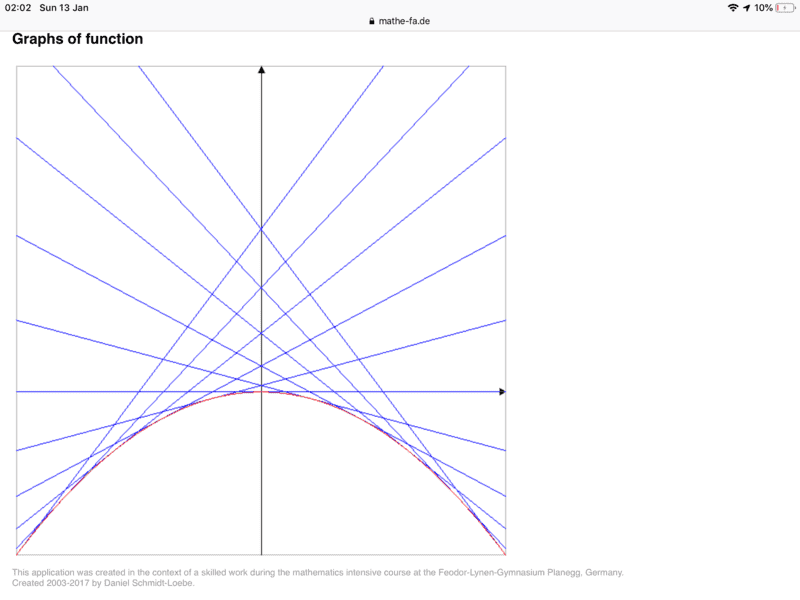# Failure of uniqueness for this first-order differential equation

1. Jan 9, 2019

### chwala1. The problem statement, all variables and given/known data
how do we establish failure of uniqueness on this first order differential equation
$y(x)= x y'+(y')^2$

2. Relevant equations

3. The attempt at a solution

general solutions are $y= cx^2+c^2$ where c = constant and
$y= -0.25x^2$
$-0.25x^2+cx+4c^2=0$
$x= -2c ⇒ y=-c^2$ for failure of uniqueness the two solutions intersect at the above points....any other alternative methods?

Last edited: Jan 9, 2019
2.3. Jan 10, 2019

### Mark44### Staff: Mentor

The above is not a solution.
$y = cx^2 + c^2 \Rightarrow y' = 2cx$
Substituting into the original diff. eqn: $x y' + (y')^2 = 2cx^2 + (2cx)^2 = 2cx^2 + 4c^2x^2 \ne cx^2 + c^2 = y$

4. Jan 10, 2019

### Ray VicksonI am confused. Does the symbol $y(x)$ on the left mean you are writing $y$ as a function of $x$ in that one place, but not in any other places on the right? Or, do you mean $y \times x?$. In other words, do you mean
$$(1): \; \left( \frac{dy(x)}{dx}\right)^2 + x \frac{dy(x)}{dx} = y(x)\; \Leftarrow ?$$
or do you mean
$$(2): \;\left( \frac{dy}{dx}\right)^2 + x \frac{dy}{dx} = xy\; \Leftarrow ?$$ Note that (2), when written out fully, would read as
$$\left( \frac{dy(x)}{dx} \right)^2 + x \frac{dy(x)}{dx} = x y(x)$$

5. Jan 10, 2019

### velaStaff Emeritus
It's a typo. The differential equation has two solutions: $y_1 = cx + c^2$ and $y_2 = -(x/2)^2$.

6. Jan 10, 2019

### chwala$y(x)$ simply means that $y$ is a function of $x$

7. Jan 10, 2019

### chwalait is a solution...

8. Jan 10, 2019

### chwalamy interest on this question was to check whether the ordinary differential equation satisfies a failure on the uniqueness ....theorem

9. Jan 10, 2019

### Mark44### Staff: Mentor

My work above proves that what you posted as a solution is NOT a solution to the differential equation you posted. Is there something you don't understand in what I wrote?

As @vela said, maybe you erred in what you wrote.

10. Jan 10, 2019

### Mark44### Staff: Mentor

The theorems I'm familiar with, such as Cauchy-Lipschitz and others, are about differential equations with initial conditions, and whether such an initial value problem has or doesn't have a unique solution. What theorem are you using to determine whether a DE has a unique solution?

11. Jan 10, 2019

### chwalai indicated that its a general solution to the problem, just correct me where i am wrong, i am still learning. When you talk of theorem , i am using the understanding that a failure of uniqueness would imply that the two solutions would intersect at some point which i indicated as $(x,y) = (-2c, c^2)$ otherwise a unique solution would not intersect with any other solution.

12. Jan 10, 2019

### chwala$(x,y) = (-2c, -c^2)$ sorry for the typo, this is where the two general solutions intersect.

13. Jan 11, 2019

### velaStaff Emeritus
So what exactly is your question?

14. Jan 11, 2019

### chwalanot so fast... first you need to confirm whether the solutions i indicated are the general solutions to the problem. Now my question was, how does one show that there is failure of uniqueness in the ordinary differential equation? further are there any other alternative ways? i was able to show that the two solutions intersect, hence failure of uniqueness.

15. Jan 11, 2019

### Mark44### Staff: Mentor

No, that's not our job -- this is your problem.

You told us of a differential equation: $(y')^2 + xy = y$
And told us that $y_1 = cx^2 + c^2$ and $y_2 = -\frac {x^2}2$ were general solutions. @vela pointed out that the first equation should be $y_1 = cx + c^2$.

You have confused me, and possibly @vela, with this question. A differential equation, without an initial condition, generally has an infinite number of solutions. In short, a solution to a first-order differential equation is not unique.

As a simple example, consider $y' = 2$. Solving this DE is simple: we get $y = 2x + C$, where C is an arbitrary constant. Notice that every solution goes through the point (0, 0). However, if an initial value is stated, such as y(0) = 1, the the unique solution is $y = 2x + 1$.

Most often when someone is talking about the uniqueness of a differential equation, they are considering an initial value problem: a differential equation and a set of initial conditions; i.e., points that are on the graph of the solution. In these types of problems, there are theorems

As near as I can tell, the question you should be asking is "why are there two solutions to this differential equation?" and not "show that there is failure of uniqueness". If your textbook has problems that concern existence and uniqueness of solutions of diff. equations, it will probably also provide some theorem, such as Cauchy-Lipschitz or Picard-Lindelof. Take a look in your book and see if these are mentioned. If not, look for a section that talks about existence and uniqueness.

I don't see why two solutions intersecting has anything to do with this problem.

16. Jan 12, 2019

### chwalai have read your comments, let me do more reading on this..though my question was more specific to the differential equation indicated...but i see your point .regards

17. Jan 12, 2019

### MidgetDwarfYou're question was not clear nor specific. You were asked to provide what Uniqueness theorems you were using. In other words, what theorems of uniqueness does you're textbook have up to the point were you're question can be found. As Mark pointed out, read you're textbook and get back to us.

18. Jan 12, 2019

### chwalaits not a textbook question, rather a differential equation in one of my past papers...otherwise i have understood his point and english is a foreign language to me, kindly understand that its not my native language.

19. Jan 12, 2019

### chwalaare there theorems on uniqueness or properties that would define a differential equation?my understanding is that uniqueness, existence and continous dependence properties are the defining factors for differentiability of functions...

20. Jan 12, 2019

### Mark44### Staff: Mentor

Which presumably would be tied to some section in the textbook you were using.

No. A differential equation is just an equation involving one or more derivatives of some function together with the function itself and possibly terms involving an independent variable.

No. A function is differentiable at a point $x_0$ in its domain if the following limit exists.
$\lim_{h \to 0} \frac{f(x_0 + h) - f(x_0)}{h}$

21. Jan 12, 2019

### epenguinYou do now need to go to a mathematical textbook. What you have come across now is that your first solution is the family of curves ( straight lines in this case case) for different arbitrary constant, c, that constitutes the general solution to the d.e.Almost visible here, foreshadowed, is the envelope of these curves which is your second solution, a parabola, drawn in red below. At every point on this curve it is tangent to a solution curve. It therefore obeys the differential equation at that point. Things like this are called the singular solution.It does not depend on any arbitrary constant,I do not know these unicity therorems or how they deal with things like this (but I would guess they define them away).They are not exactly different solutions to the general one, just a set of its solution points

I always found these peculiar singular solutions amusing, and sometimes played with them, though like a lot of differential equation theory and methods I forget them between one time and another. I presume they do not come up in any physical d.e.s and if a system found itself on a singular solution it wouldn't know which way to go then. (But as soon as you say anything like that you often find out that instead it is a flourishing research area.)

Last edited: Jan 13, 2019